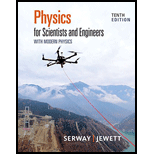# A point charge +2 Q is at the origin and a point charge − Q is located along the x axis at x = d as in Figure P22.11. Find a symbolic expression for the net force on a third point charge + Q located along the y axis at y = d . Figure P22.11### Physics for Scientists and Enginee...

10th Edition
Raymond A. Serway + 1 other
Publisher: Cengage Learning
ISBN: 9781337553292

#### Solutions

Chapter
Section### Physics for Scientists and Enginee...

10th Edition
Raymond A. Serway + 1 other
Publisher: Cengage Learning
ISBN: 9781337553292
Chapter 22, Problem 11P
Textbook Problem
23 views

## A point charge +2Q is at the origin and a point charge −Q is located along the x axis at x = d as in Figure P22.11. Find a symbolic expression for the net force on a third point charge +Q located along the y axis at y = d.Figure P22.11To determine

The symbolic expression for the net force on a third point charge +Q located along the y=d .

### Explanation of Solution

Given Info: The first point charge +2Q located at the origin and the second point charge Q located along x -axis at x=d .

The given diagram is shown below.

Figure (1)

The force acting on +Q charge due to the +2Q charge is,

F1=K(2Q)(Q)d2j^=2KQ2d2j^

Here,

2Q is the first point charge.

Q is the third point charge.

d is the distance between point charges.

K is the constant.

The direction of force is along the y -axis then the unit vector is introduced along the y direction.

The expression for the distance between second and third point charges is given as,

d2=d2+d2=2d2d=2d

The electrical force acting on the +Q charge due to Q Charge is,

F2=K(Q)(Q)(d)2((cosθ)i^+(sinθ)j^)

The angle between the second point charge and third point charge is 45° .

Substitute 45° for θ and 2d for d in the above expression

### Still sussing out bartleby?

Check out a sample textbook solution.

See a sample solution

#### The Solution to Your Study Problems

Bartleby provides explanations to thousands of textbook problems written by our experts, many with advanced degrees!

Get Started

Find more solutions based on key concepts
What If? The block of mass m = 200 g described in Problem 43 (Fig. P8.43) is released from rest at point , and ...

Physics for Scientists and Engineers, Technology Update (No access codes included)

What is the defining feature of a synovial joint?

Human Biology (MindTap Course List)

The maturation of sperm takes 7080 days. ______ True ______ False

Nutrition Through the Life Cycle (MindTap Course List)

In the following diagram, designate each daughter cell as diploid (2n) or haploid (n).

Human Heredity: Principles and Issues (MindTap Course List)

Worldwide, of those who die each year from starvation and related illnesses are children, a three-fourths one-t...

Nutrition: Concepts and Controversies - Standalone book (MindTap Course List)